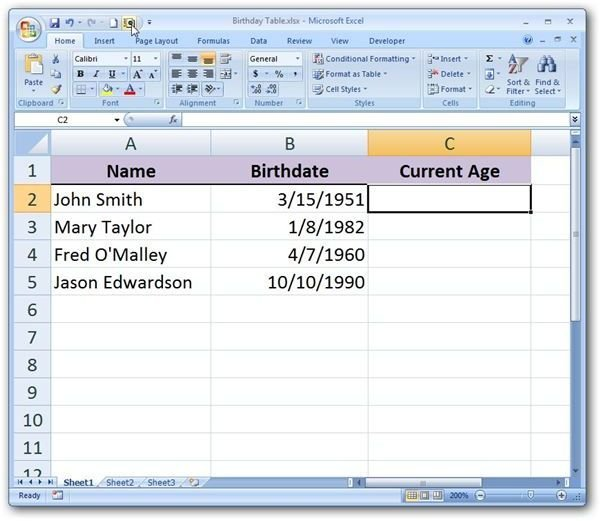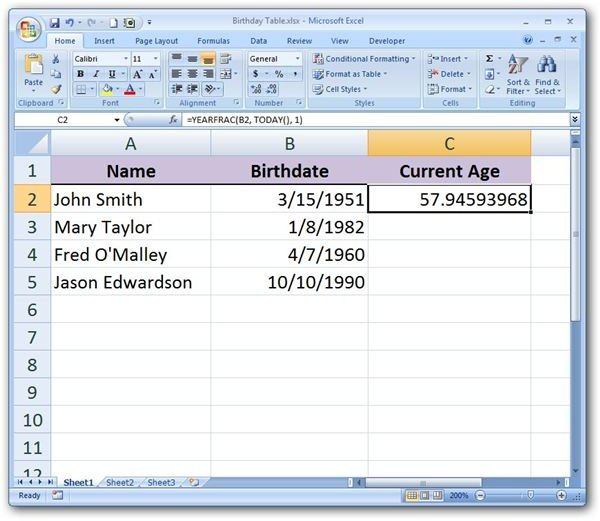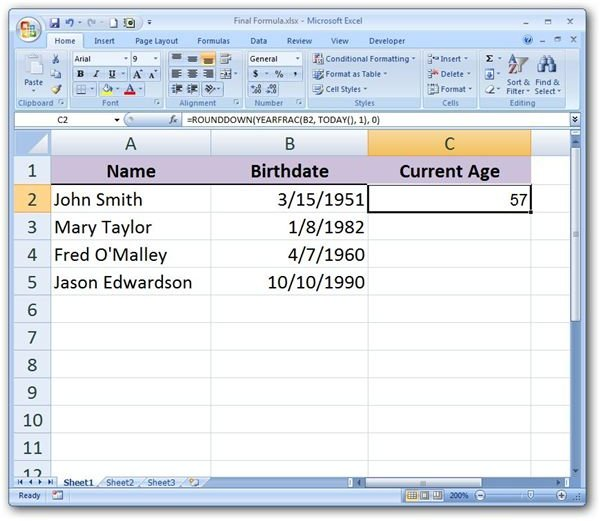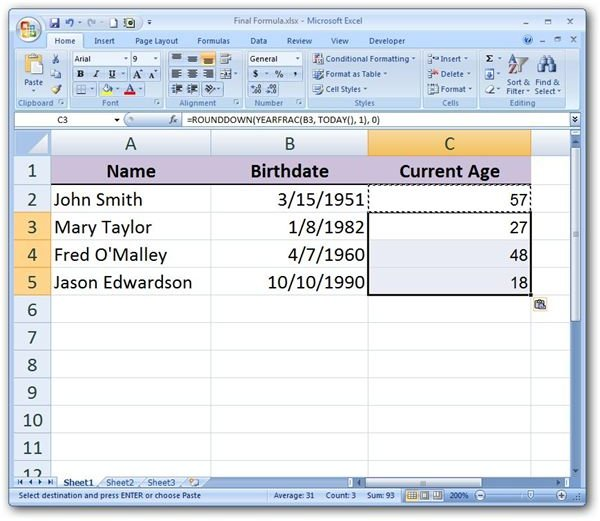# How to Calculate a Person’s Current Age in Microsoft Excel

Page content

## The Functions We’ll Need

Suppose we have a list of birth dates in Excel and we want to create a function that will calculate each person’s age (in years) as of today’s date. There are several ways to do this, but one of the most straightforward methods involves combining the YEARFRAC function with the TODAY() function and then applying ROUNDDOWN to the result so that we only include the number of years in the final answer.

If that’s starting to sound complicated, don’t worry – it’s really not that bad at all. We’ll walk through the process step by step and include formulas that you can cut and paste into your own Excel spreadsheet.

## Coming Up With the Formula

First, we’ll start with a list of birthdates. (Click any image for a larger view.)In this example, we’ll create a formula for Column C of the table that will calculate the current age based on the given birth date and today’s date.

Next, recall that the YEARFRAC function has the following syntax. (For more details on this function, see Microsoft Excel’s YEARFRAC Function.)

YEARFRAC(start_date, end_date, basis)

In this instance, we would want to use a basis of 1 since we want calculations based on the actual number of days in the year. Our start_date would be the birth date that is found in Column B of the table. Since we want to use the current date as our end_date, we will substitute that argument with the TODAY() function.

All of this information translates into the following formula when applied to Row 2 of the table.

=YEARFRAC(B2, TODAY(), 1)This formula would be fine if we wanted to include the partial year information for the current age, but all we really want here is the integer portion of the year. To obtain this, we can incorporate the ROUNDDOWN function into the formula. The basic syntax for this function is

ROUNDDOWN(number, num_digits)

where number represents the item we are rounding down and num_digits­ is the number of decimal places we want to include.

In our example, we want to round the result down and only keep the integer portion of the number, so num_digits would be 0. The number itself would be our formula from above. So, our new formula is the following.

=ROUNDDOWN(YEARFRAC(B2, TODAY(), 1), 0)After you’ve entered this formula into cell C2 of the table, you can cut and paste it into the remaining cells of the column or use Excel’s column fill tool.Note: If today is a person’s birthday, this function will round down their age so it will not display the correct age until the day after. For another method to do this, try the formula: =DATEDIF(start_date, end_date, basis); Example: =DATEDIF(B2,TODAY(),“y”).

For more tips and tricks, take a look at the other items in Bright Hub’s collection of Microsoft Excel user guides and tutorials. Find out how to use the various lookup and reference functions, learn about constructing charts and graphs, read up on data validation tools, and more. Additional items are being added on a regular basis, so be sure to check back often.